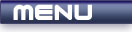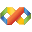Home Latest News Archive Tutorials C++ 2 3 4 Win32 Java OpenGL GLUT OpenGL ES 2 3 4 DirectX VB.NET HTML Photoshop 3dsmax Forums Projects Modelling Links About Us Contact Search Recent Tutorials C / C++ Win32 OpenGL OpenGL ES GLUTTutorials > C++ > Advanced Output

### Introduction

You saw in the last tutorial that you were able to print out variables using the cout object. You may found that you are wanting to create a C only program where you will only be using the printf function. This tutorial will show you how to print out variables with the printf command.

It is also possible to set formatting for C++ output using the cout object.

We will also go through various escape characters that you can use when printing out strings. These are characters consisting of a \ and a character. You were introduced to the \n escape character in previous tutorials.

Contents of main.cpp :

The iomanip include is used for the C++ formatting functions. It is not needed for the C functions.

```#include <stdio.h>
#include <iostream>
#include <iomanip>
#include <stdlib.h>

using namespace std;

int main()
{
/************************/
// Variable Declarations
/************************/
char c = 'a';
short s = 56;
int i = 4500;
long l = 500000;
float f = 45.34f;
double d = 233423.2362;
```

In the past, you have used the printf function in the following way :

printf(char * output);

You might not know what a char * is. We will explain this later but for now you will only need to know that this can be used to store a string of characters. The string for this function can also include various format specifications as shown below :

printf(char *format, arguments... , ... , ...);

You can use this method to print variables out to the console.

Many format specifications can be shown in the table below :

 Type Specification Character %c String %s Whole Number %d Floating point Number %f Hexadecimal Format %X Octal Format %o Unsigned Number %u

```	/***********/
// C Output
/***********/
printf("C Output\n-------------------------\n");
// Characters / Strings
printf("Character 1 : %c Character 2 : %c\n", c, 't');
printf("String : %s\n", "Hello");

// Numbers
printf("Short : %d\n", s);
printf("Integer : %d\n", i);
printf("Long : %d\n", l);

// Floating point Numbers
printf("Float : %f\n", f);
printf("Double : %f\n", d);
```

You can specify how what precision a decimal number should have, ie. how many decimal places are used to round off. This is done by placing a .n between the % and the f where n is the number of decimal places you are interested in eg. %.3f prints out a decimal number, formatting it with 3 decimal places.

The unsigned number printed below will end up being extremely large as the %u enforces the use of a positive number. This results in the number being 500 away from the maximum possible integer value as negative numbers wrap around to the highest possible unsigned integer value.

```	// Number formatting
printf("Float with 2 Decimal digits : %.2f\n", d);
printf("Decimal : %d, Hex : %X, Octal : %o\n", i, i, i);
printf("Unsigned number : %u\n", -500);
```

When using format specifications, you may also insert a number between the % and the letter. This will make the variable being printed out fill a specific amount of space. If the number is less than the space needed, the space will be expanded to allow the variable to be displayed eg. specifying %5c will print out a character but this character will be placed with 4 spaces in front of it, hence taking up a total of 5 spaces. This is useful for creating columns for your output.

The \0 character is a null character which means that it is basically nothing. This can be used to just created a space.

```	// Character Formatting
printf("Hello%4cHello%10cHello%7s\n", '\0', '\0', "String");

printf("\n");
```

A similar method is available for C++ to create columns. This is achieved by using the setw method while outputting data. It takes one argument which is the amount of space you want taken up by the following data.

```	/*************/
// C++ Output
/*************/
cout << "C++ Output" << endl << "------------------------------" << endl;
// Width of Display Field
cout << setw(20) << "Block 1" << setw(10) << "Block 2" << endl;
```

This text is alway right-aligned. We may want it to be left aligned and therefore we can set the format for all outputted text. This can be done using the setf method. It takes various ios flags as arguments. ios::left is an example of such a flag. Activating this flag will allow all data to be left-aligned.

```	cout.setf(ios::left);
cout << setw(20) << "Block 1" << setw(10) << "Block 2" << endl;
```

As the setf method enables a flag for all further output, you may want to reset certain flags. This can be achieved by using the unsetf method as shown below.

```	cout.unsetf(ios::left);
cout << setw(20) << "Block 1" << setw(10) << "Block 2" << endl;
```

You may have the need to fill the spaces created by setw with a character other than a space. This can be achieved by using the setfill method. The method takes one parameter which is the character you would like to use to fill the spaces.

```	// Fill
cout << setw(20) << setfill('#')
<< "Block 1" << setw(10) << "Block 2" << endl;
```

To set the precision for decimal numbers in C++, you could use the setprecision method which takes one parameter, a number specifying the number of decimal places used for precision.

Scientific notation is used by default, so you may want to change this default behaviour by activating the ios::fixed flag. Try leaving this line out to see the difference.

```	// Precision
cout.setf(ios::fixed);
cout << setprecision(2) << d << endl;

printf("\n");
```

There are many escape characters available for use in strings. A few can be shown in the following table below :

 Character Description \n Newline \t Tab \a Alert (beep) \" Quote \r Carriage Return

```	/********************/
// Escape Characters
/********************/
printf("Escape Characters\n---------------------------");
printf("\tTabbed text\n"); // tab
printf("Alarm\a\n"); // alarm
printf("Quotes : \"Hello\"\n"); // quotes

system("pause");

return 0;
}```

Congratulations. You should now be able to effectively customize your output, giving you greater flexibility in displaying your information. Many functions have been covered in this tutorial, so experiment yourself to experience the effects yourself.

Please let me know of any comments you may have : Contact Me

 Source Files :< Tutorial 05 - Data Types Tutorial 07 - Arrays >